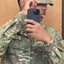MathematicsOpenStudy (anonymous):

An open rectangular box with square base is to be made from 48 ft^2 of material. What dimensions will result in a box with the largest possible volume?OpenStudy (bahrom7893):

bear with me.. solvingOpenStudy (bahrom7893):

Surface area is area of the bottom: x^2 + 4 sides: 4*xy Surface area = 48ft^2 = x^2 + 4xy Volume is x^2*y: V = x^2 * yOpenStudy (bahrom7893):

Solve for y in the surface area: 48 = x^2 + 4xy 48-x^2 = 4xy (48-x^2)/4x = yOpenStudy (bahrom7893):

y = (48 - x^2) / 4x V = x^2 * y = x^2 * (48 - x^2) / 4x = x ( 48-x^2) / 4OpenStudy (bahrom7893):

Simplify a bit: V = 48x/4 - x^3/4 = 12x - x^3/4 Take the derivative and set it equal to 0: V ' = 12 - 3x^2 / 4 = 0OpenStudy (bahrom7893):

Solve the equation: 12 - 3x^2 / 4 = 0 -3x^2/4 = -12 x^2/4 = 4 x^2 = 4*4 = 16OpenStudy (bahrom7893):

x = + or - 4, but in this case it's a plus because the edge of the box cannot be negative.. anyway let's double check if it's min or max. To do so, take the 2nd derivative: V" = -6x / 4 = - 3x / 2. Which is positive if x = -4 and negative if x = 4. Maximum occurs when 2nd derivative is negative therefore x = 4 is maxOpenStudy (bahrom7893):

Okay so now that you have x = 4, find y: y = (48 - x^2) / 4x y = (48 - 16) / 16 = 32/16 = 2 So dimensions are: The sides of the base must be equal to 4, and the height of the box must be 2. In which case the volume = area of the base * height: V = 4^2 * 2 = 16 * 2 = 32OpenStudy (bahrom7893):

Hope this helped =)OpenStudy (bahrom7893):

by the way units are: x = 4ft y = 2ft V = 32ft^3

Latest QuestionsTrickjoker: What happened to the Scotty chat AI bot?
13 minutes ago 0 Replies 0 MedalsTootie14: Real life poem how do y'all like it I found someone that i think will make me happy but is it worth trusting.
59 minutes ago 6 Replies 3 MedalsAquarytheALPHA: WANNA ZOOM?
2 hours ago 11 Replies 0 Medalsbootyfulgirl: @Supreme01 helppppp
4 hours ago 26 Replies 0 Medalsaxie: How to use bass boosted music on windows! (tutorial)
6 hours ago 11 Replies 2 MedalsCRYSTALC: https://drive.google.com/file/d/1JYWSUWpXf8jVsJChwkz2Y023haObCuy5/view?usp=sharin
3 hours ago 7 Replies 3 MedalsForeverangel: I really need help please. What is the width of the pond, East to West? Round answer to nearest foot.
9 hours ago 1 Reply 0 MedalsForeverangel: I really need help please this is almost due and I can't figure it out. Amplitude
10 hours ago 16 Replies 1 Medal441204: https://www.bandlab.com/2004_diorq/dior-q-loosing-you-prod-billygotitbumpin-44392
7 hours ago 5 Replies 1 Medalalanhernandez: (02.01)Polygon ABCD slides 4 units left and 3 units up on the coordinate plane. I
9 hours ago 1 Reply 0 Medals